php 2个多维数组找出不同数值

Luffy666 发布于 2013/05/03 09:11

年薪达不到25W大数据工程师、拿不到Offer全额退款->>>array=（

0=>array(

a=>'18900',

b=>'14500',

),

1=>array(

a=>'18900',

b=>'14500',

)

array=（

0=>array(

a=>'18900',

b=>'14500',

),

1=>array(

a=>'18901',

b=>'14501',

)

a=>'18901',

b=>'14501',

0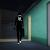array_diff 只是针对两个或两个以上的一维数组的。

0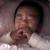0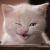0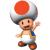http://www.93337.com/ism/

0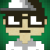\$arrA = array(
array('a' => '18900', 'b' => '14500'),
array('a' => '18900', 'b' => '14500'),
);

\$arrB = array(
array('a' => '18900', 'b' => '14500'),
array('a' => '18901', 'b' => '14501'),
);

function foo(\$arr, &\$rt) {
if (is_array(\$arr)) {
foreach (\$arr as \$v) {
if (is_array(\$v)) {
foo(\$v, \$rt);
} else {
\$rt[] = \$v;
}
}
}
return \$rt;
}
\$rtA = array();
\$rtB = array();
foo(\$arrB, \$rtB);
foo(\$arrA, \$rtA);
var_dump(array_diff(\$rtB, \$rtA));